## Example Questions

### Example Question #866 : Algebra

Which of the following is a value ofthat satisfies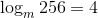?Explanation:

When you have a logarithm in the form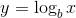,

it is equal to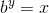.

Using the information given, we can rewrite the given equation in the second form to get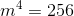.

Now solving forwe get the result.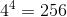### Example Question #867 : Algebra

Solve for: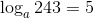Explanation:

When you have a logarithm in the form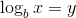,

it is equal to.

We can rewrite the given equation as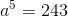Solving for, we get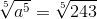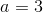.

### Example Question #868 : Algebra

Solve for: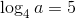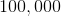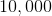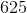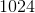Explanation:

When you have a logarithm in the form,

it is equal to.

We can rewrite the given equation as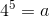Solving for, we get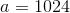.

### Example Question #869 : Algebra

Converting exponents to rational numbers often allows for faster simplification of those numbers.

Which of the following is incorrect? Convert exponents to rational numbers.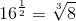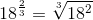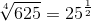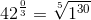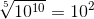Explanation:

To identify which answer is incorrect we need to do each of the conversions.

First lets look at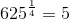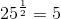.

Therefore this conversion is true.

Next lets look at. For this particular one we can recognize that anything raised to a zero power is just one therefore this conversion is true.

From here lets look at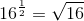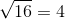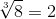Thus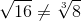. Therefore this is an incorrect conversion and thus our answer.

### Example Question #870 : Algebra

Sometimes, seeing rational numbers makes it easier to understand an equation.

Convert the following into a rational number or numbers: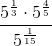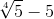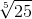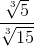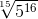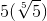Explanation:

The rule for converting exponents to rational numbers is: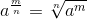.

Even with this, it is easier to work the problem as far as we can with exponents, then switch to rational expression when we run out of room: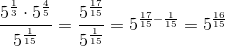At last, we convert, and obtain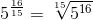.

Thus,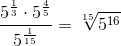.